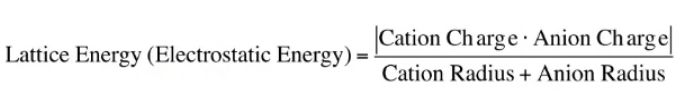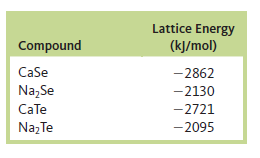# Problem: Rationalize the following lattice energy values:

###### FREE Expert Solution

The relationship of the charge and the atomic radius of the ions to the lattice energy can be expressed by the equation below.From this, we can say that the effect of charge must be higher compared to the radius because the effects are multiplicative compared to the additive effect of the radius.

Also, take note that charges are always whole numbers and we account for the absolute value so multiplying them would always get a product that would cause an increase.

80% (471 ratings)###### Problem Details

Rationalize the following lattice energy values: